GROUP THEORY SYMBOL

# GTDensityOfStatesRSGTDensityOfStatesRS[Hamiltionian,parameters]
is used to calculate the density of states of a real space Hamiltionian. Instead of a Hamiltionian a list of eigenvalues can be used insead.

## DetailsDetails

• If the real space Hamiltonian is an N×N matrix, N eigenvalues will be calculated. The density of states will be replaced by a sum of Gaussian located at the eigenvalues, having a width σ. The integrated density of states can be calculated as well.
• Instead of the Hamiltonian a list of eigenvalues can be used. This allows the folllowing scheme of calculation. The Hamiltonian can be con structed by means of GTTbHamiltonianRS. This real space Hamiltonian can be exported to a FORTRAN subroutine by means of GTTbRSToFortran. The eigenvalues can be calcuated with optimized routines from nuumerical libraries.
• The vector parameters contains the following quantities:
• parameters = {nbas, nat, emin, emax, ne, σ, scale}
•  nbas number of atoms in the basis nat number of atoms in the cluster [emin,emax] energy intervall for calculation of density of states ne number of energy points used to calculate the density of states σ width of the Gaussians (if σ = 0 the energy step width is used) scale The whole DOS can be scaled by the factor scale. This is sometimes helpful for comparisons.
• The following options can be given:
•  FrameLabel {"Energy", "DOS"} Option of ListPlot GOFermiEnergy 0 Calculates the Fermi energy GOPlotDos "DOS" Plots density of states or integrated DOS or both GOStore 0 Stores the DOS, if a file name is given PlotLabel "Density of states" Option of ListPlot PlotRange All Option of ListPlot PlotStyle RGBColor[1, 0, 0] Option of ListPlot
• See: W. Hergert, M. Geilhufe, Group Theory in Solid State Physics and Photonics. Problem Solving with Mathematica, chapter 9.7.3.

## ExamplesExamplesopen allclose all

### Basic Examples  (1)Basic Examples  (1)

 In:=In:=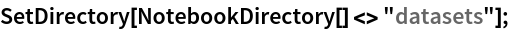The density of states of GaAs is used as an example. The DOS is first calculated in k-space. The Hamiltonian for the calculation is already prepared. The Hamiltonian is prepared with a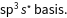In:=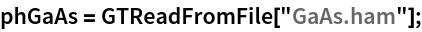The density of states in k-space can be calculated now.

 In:=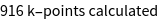Out=The Hamiltonian in real space has the dimension 7090x7090. The corresponding cluster consists of 709 Ga and 709 As atoms. This real space Hamiltonian is also already prepared:

 In:=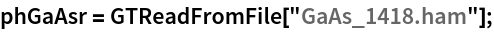The density of states can be calculated.

 In:=Out=In:=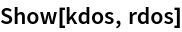Out=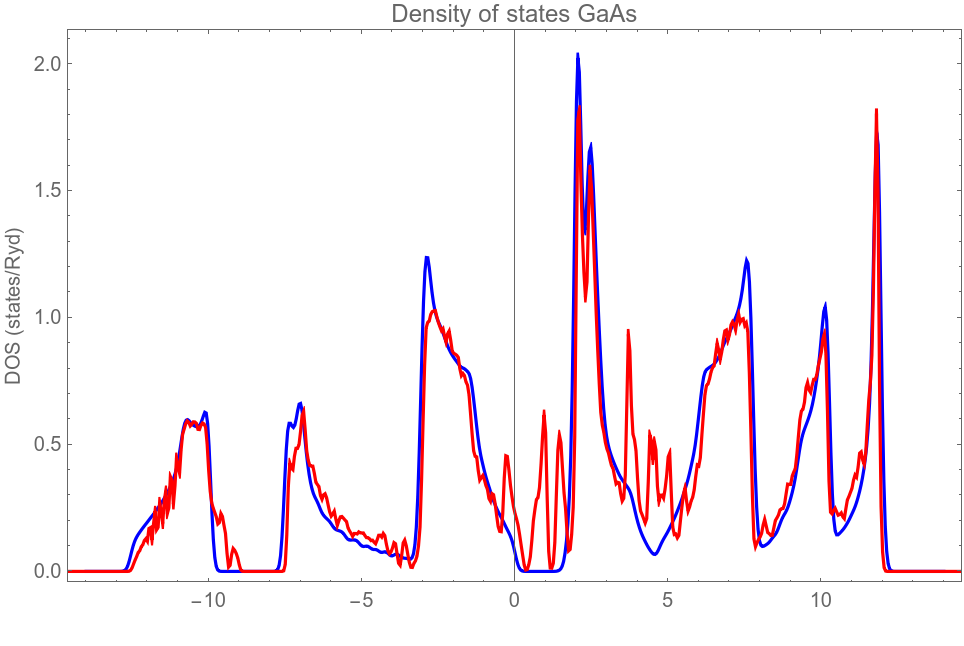The comparison of both DOS calculations shows, that due to the surface of the cluster surface states appear in the band gap in the real space calculation.

To demsonstrate, that instead of the Hamiltonian also a list of eigenvalues can be provided for the DOS calculation, we calculate the eigenvalues from the real space Hamiltonian.

 In:=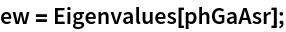In:=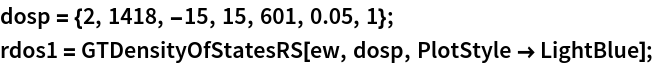In:=Out=Obviously the results ar identical.

### Options  (3)Options  (3)

GTDensityOfStatesGTDensityOfStatesPlotGTFindStateNumbersGTPlotStateWeightsGTTbHamiltonianRSGTTbRealSpaceMatrixGTPartialDOSGTBandsDOSPlot